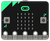## How do I get the input data from the accelerometer to transmit it to another microbit?

I need to display the accelerometer movement on the LEDs of another microbit by tilting the microbit.

#### Question relating to:The BBC micro:bit is a pocket-sized, codable computer that allows anyone to get creative with technology. Made possible through a major partnership with 31 organisations, a micro:bit has been given …

##### 2 years, 3 months ago.

Hi Affiq,

I would take a look at the following resources:

Please let me know if you have any questions!

- Jenny, team Mbed

Hi Jenny,

Thanks for answering. I've done that bit but now I need to send a string value from one microbit to the other one. I'm having trouble trying to convert the string to int to display the accelerometer data on the other microbit. Would appreciate it any help given.

Thanks, Affiq

posted by Affiq Arsat 27 Mar 2018

Hi again,

You can convert a character array to int with the following code:

```char *myStr = "1234";
int myInt = atoi(myStr);
```

- Jenny, team Mbed

posted by Jenny Plunkett 27 Mar 2018

This also may be helpful: https://os.mbed.com/questions/2910/Convert-string-to-int/

posted by Jenny Plunkett 27 Mar 2018

Hi again, This is my code for the transmitter.

```#include "MicroBit.h"
#include <string>

MicroBit uBit;
Serial pc(USBTX, USBRX);
//
// Scales the given value that is in the -1024 to 1024 range
// int a value between 0 and 4.
//
int pixel_from_g(int value)
{
int x = 0;

if (value > -750)
x++;
if (value > -250)
x++;
if (value > 250)
x++;
if (value > 750)
x++;

return x;
}

int main()
{
// Initialise the micro:bit runtime.
uBit.init();
//
// Periodically read the accelerometer x and y values, and plot a
// scaled version of this ont the display.
//
while(1)
{
int acc_x = uBit.accelerometer.getX();
int acc_y = uBit.accelerometer.getY();

int x = pixel_from_g(acc_x);
int y = pixel_from_g(acc_y);
uBit.display.image.clear();
uBit.display.image.setPixelValue(x, y, 255);

//char buf1;
ManagedString s1(acc_x);
ManagedString s2(acc_y);
ManagedString s = s1 + s2;
//std::sprintf (buf1, "%s",acc_x );

pc.printf("tx\r\n");
uBit.sleep(1000);
}
}
```

And this is my code for the receiver.

```#include "MicroBit.h"
#include <stdlib.h>
#include <stdio.h>
#include <string.h>
#include <string>

using namespace std;
Serial pc(USBTX, USBRX);

MicroBit uBit;

//
// Scales the given value that is in the -1024 to 1024 range
// int a value between 0 and 4.
//

int pixel_from_g(int value)
{
int x = 0;

if (value > -750)
x++;
if (value > -250)
x++;
if (value > 250)
x++;
if (value > 750)
x++;

return x;
}

void onData(MicroBitEvent)
{
int num = atoi(b.toCharArray());

//int x = pixel_from_g(acc_x);
//int y = pixel_from_g(acc_y);

pc.printf("num = %d", num);

}

int main()
{
// Initialise the micro:bit runtime.
uBit.init();

char str1[] = "123";
int num = atoi(str1);
pc.printf("rx\r\n");

while(1)
{

uBit.sleep(1000);
}
}
```

I need to send the accelerometer values from the transmitter to the receiver. I'm currently stuck here. Thanks for all the help so far.

Thanks, Affiq

posted by Affiq Arsat 28 Mar 2018